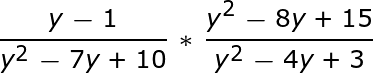# How to Multiply and Divide Rational Expressions

An error occurred trying to load this video.

Try refreshing the page, or contact customer support.

Coming up next: Multiplying and Dividing Rational Expressions: Practice Problems

### You're on a roll. Keep up the good work!

Replay
Your next lesson will play in 10 seconds
• 0:06 Polynomial Rational…
• 1:10 Example #1
• 3:44 Example #2
• 5:46 Example #3
• 7:20 Lesson Summary
Save Save

Want to watch this again later?

Timeline
Autoplay
Autoplay
Speed Speed

#### Recommended Lessons and Courses for You

Lesson Transcript
Instructor: Kathryn Maloney

Kathryn teaches college math. She holds a master's degree in Learning and Technology.

Multiplying and dividing rational polynomial expressions is exactly like multiplying and dividing fractions. Like fractions, we will reduce. With polynomial expressions we use factoring and canceling. I also give you a little mnemonic to help you remember when you need a common denominator and when you don't.

## Multiplying and Dividing Rational Expressions

A polynomial rational expression is a fraction containing polynomials. Example of a rational expression: (r - 4) ÷ (r² - 5r + 6)

Today we're going to look at multiplication and division of rational polynomial expressions. Remember how we multiplied fractions? We multiplied straight across the fractions. The same rule applies to rational polynomial fractions. It's just like 1/2 * 3/5, where we multiply straight across to get 3/10.

Division of fractions requires use to 'flip' the second fraction. You got it! That rule also applies to rational fractions. It's just like with 1/9 ÷ 2/7, where we flip the second fraction and change it to multiplication, so we have 1/9 * 7/2. Multiply straight across, and we get 7/18.

So is it really that easy? Almost. We need to add a couple new steps, but they aren't too bad.

## Example #1First, we need to get the polynomials in simplified form. That means factoring. Let's review factoring. Let's look at y² - 7y + 10. We're looking for multiples of 10 that add to -7.

-2 * -5 = 10, and -2 + -5 = -7. So y² - 7y + 10 factors into (y - 2)(y - 5).

How about y² - 8y + 15? Well, -3 * -5 = 15, and -3 + -5 = -8, so y² - 8y + 15 factors into (y - 3)(y - 5).

And then y² - 4y + 3 factors into (y - 1)(y - 3).

So it turns out we have:

((y - 1) ÷ ((y - 5)(y - 2))) * (((y - 3)(y - 5)) ÷ ((y - 1)(y - 3)))

The second new step is reducing, or what I like to call slashing! You can only reduce from top to bottom - never reduce from side to side! Once you have 'slashed' all of the like terms from the top and bottom, we multiply straight across. Don't multiply anything we slashed!

We can cancel (y - 5) over (y - 5) and (y - 1) over (y - 1).

Are you asking yourself, why we can cancel? Well, we know that 4 ÷ 4 = 1; then (y - 3) ÷ (y - 3) also equals 1, so we slash those, too! Once we've 'slashed' all of the like terms from the top and bottom, we multiply straight across. Don't multiply anything we slashed! Why? Because those are now 1s!

It turns out that ((y - 1) ÷ (y² - 7y + 10)) * ((y² - 8y + 15) ÷ (y² - 4y + 3)) = 1 ÷ (y - 2).

## Example #2

Let's look at ((r - 4) ÷ (r² - 5r + 6)) ÷ ((r - 3) ÷ (r² - 6r + 9)).

First, we need to get the polynomials in simplified form, and that means factoring. Let's review again factoring. Let's look at r² - 5r + 6. We're looking for multiples of 6 that add to -5.

-3 * -2 = 6, and -3 + -2 = -5. So r² - 5r + 6 factors into (r - 3)(r - 2).

How about r² - 6r + 9? Well, -3 * -3 = 9 and -3 + -3 = -6, so r² - 6r + 9 factors into (r - 3)(r - 3).

Our expression with simplified polynomials now looks like:

((r - 4) ÷ ((r - 3)(r - 2))) ÷ ((r - 3) ÷ ((r - 3)(r - 3)))

To unlock this lesson you must be a Study.com Member.

### Register to view this lesson

Are you a student or a teacher?

#### See for yourself why 30 million people use Study.com

##### Become a Study.com member and start learning now.
Back
What teachers are saying about Study.com

### Earning College Credit

Did you know… We have over 200 college courses that prepare you to earn credit by exam that is accepted by over 1,500 colleges and universities. You can test out of the first two years of college and save thousands off your degree. Anyone can earn credit-by-exam regardless of age or education level.